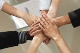# Binding Radgrid columns with child object list

GridTop achievementsRank 1
Hello,

I have a Radgrid where I an creating the columns dynamically on page_load, when databound the data shouws fine except where I created columns bound to a child object which in this case is a list of integers, those columns are created as shown below

`for` `(i = 0; i < weeks; i++)`
`                   ``{`
`                       ``AddGridColumns(Grid, ``"ChildList["` `+ i.ToString() + ``"]"``, ``"HeaderText"``);`
`                       `

`                   ``}`

`private void AddGridColumns(RadGrid Grid, string DataField,string HeaderText)`
`        ``{`
`             `
`            ``GridBoundColumn boundColumn = new GridBoundColumn();`
`            ``Grid.MasterTableView.Columns.Add(boundColumn);`
`            ``boundColumn.DataField = DataField;`
`            ``boundColumn.HeaderText = HeaderText;`
`            ``boundColumn.UniqueName = DataField;`
`  `
`        ``}`

I have confirmed that the list contains data at the respective indexes and the rest of the columns show data as expected, any help would be appreciated.

## 1 Answer, 1 is accepted

0Princy
Top achievementsRank 1
answered on 01 Oct 2013, 08:31 AM

C#:
`private` `void` `AddGridColumns(RadGrid Grid, ``string` `value, ``string` `HeaderText)`
`   ``{`
`       ``GridBoundColumn boundColumn = ``new` `GridBoundColumn();`
`       ``Grid.MasterTableView.Columns.Add(boundColumn);`
`       ``boundColumn.DataFormatString = value;       `
`       ``boundColumn.HeaderText = HeaderText;`
`       ``boundColumn.UniqueName = value; `
`   ``}`

Thanks,
Princy
Tags
GridRank 1Rank 1